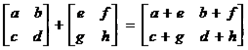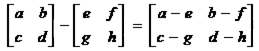## 2x2 Matrix Addition & Subtraction Calculator

 Result: A + B = A - B =

The 2x2 Matrix Subtraction & Addition Calculator to find 2x2 matrix addition and subtraction result between the two 2x2 matrices A and B.